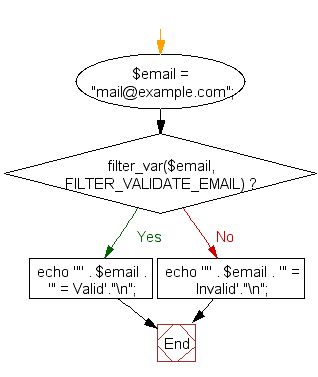﻿ PHP Exercise: Email validation - w3resource

# PHP Exercises : Email validation

## PHP : Exercise-12 with Solution

Write a simple PHP program to check that emails are valid.

Hints : Use FILTER_VALIDATE_EMAIL filter that validates value as an e-mail address.
Note : The PHP documentation does not say that FILTER_VALIDATE_EMAIL should pass the RFC5321.

Sample Solution: -

PHP Code:

``````<?php
// pass valid/invalid emails
\$email = "[email protected]";
if (filter_var(\$email, FILTER_VALIDATE_EMAIL))
{
echo '"' . \$email . '" = Valid'."\n";
}
else
{
echo '"' . \$email . '" = Invalid'."\n";
}
?>
```
```

Sample Output:

```"[email protected]" = Valid
```

filter_var() function:

Syntax:

`mixed filter_var ( mixed \$variable [, int \$filter = FILTER_DEFAULT [, mixed \$options ]] )`

filter_var() function filters a variable with a specified filter.

Parameters:

• variable: Value to filter.
• filter: The ID of the filter to apply.
• options: Associative array of options or bitwise disjunction of flags.

Flowchart:PHP Code Editor:

Have another way to solve this solution? Contribute your code (and comments) through Disqus.

What is the difficulty level of this exercise?

Test your Programming skills with w3resource's quiz.

﻿

## PHP: Tips of the Day

Mutates the original array to filter out the values specified

Example:

```<?php
function tips_pull(&\$items, ...\$params)
{
\$items = array_values(array_diff(\$items, \$params));
return \$items;
}

\$items = ['x', 'y', 'z', 'x', 'y', 'z'];
print_r(tips_pull(\$items, 'y', 'z'));
?>
```

Output:

```Array
(
 => x
 => x
)
```